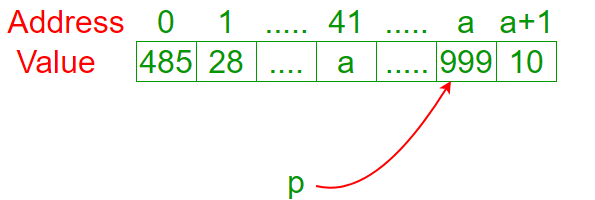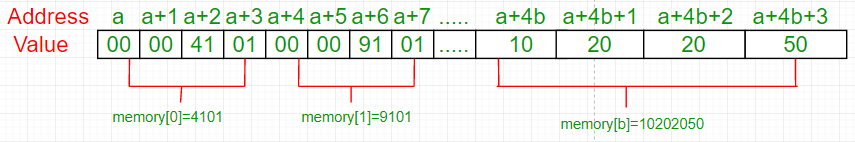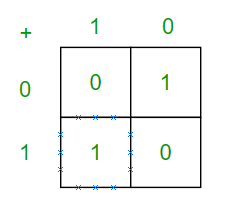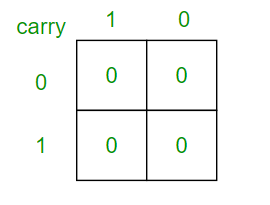# How to sum two integers without using arithmetic operators in C/C++?

Given two integers a and b, how can we evaluate the sum a + b without using operators such as +, -, ++, –, …?

Method 1 (Using pointers)

An interesting way would be:

 `// May not work with C++ compilers and ` `// may produce warnings in C. ` ` `  `// Returns sum of 'a' and 'b' ` `int` `sum(``int` `a, ``int` `b)  ` `{ ` `    ``char` `*p = a; ` `    ``return` `(``int``)&p[b]; ` `} `

Despite its awkwardness at first sight, we can easily understand what is happening. First, we created a pointer p. The value of a pointer is a memory address. In this case, the value of p is the address a.Remember: p points to position a. In that example, if we want to know the value of position a (999) we ask to *p. If we want to know the address of variable a (41), we ask to &a.

If we evaluated p[b], we’d get the value of memory in position p + b. In fact, we evaluate &p[b], witch is the same as getting address p + b without accessing its value. As p = a, &p[b] will return the address a + b.

We don’t want to return a memory address (int*). We want to return an integer (int). So, we cast &p[b] to int.

What happens if we change the type of p from char* to int*? Let me fix what I said before: When we evaluate p[b] we don’t evaluate p + b. We evaluate p + sizeof(*p) * b. Why? Imagine this example: Variables of type int occupies fours positions in the memory.This sizeof(*p) considers the quantity of positions each variable occupies in the memory.

We want to evaluate p + b. In other words, we want sizeof(*p) equals to 1. Consequently, if *p is a char, we’re happy.

Method 2 (Using bitwise operators)

Let’s look the sum’s truth table (forgive the carry for now):

Looking carefully, we notice that the sum’s and xor’s truth table are the same. It’s 1 only when the input differs.

Now, how can we detect carry? Let’s look the carry’s truth table.Looking carefully, we notice that the sum’s and xor’s truth table are the same. It’s 1 only when the input differs.

Now, how can we detect carry? Let’s look the carry’s truth table.Looking carefully one more time, we notice that the carry’s and logical and’s truth table are identicals. Now, we have to shift a & 1 to left and sum with a ^ b. However, these operations may have carries as well. No problem, just sum a ^ b with a & 1 shifted to left recursively.

 `// Returns sum of a and b using bitwise ` `// operators. ` `int` `sum(``int` `a, ``int` `b)  ` `{ ` `    ``int` `s = a ^ b; ` `    ``int` `carry = a & b; ` ` `  `    ``if` `(carry == 0) ``return` `s; ` `    ``else` `return` `sum(s, carry << 1); ` `}`

This solution has been discussed here.

Method 3 (Using printf)

Let’s remember some facts:

• The printf returns the number of characters printed successfully.
• The specifier %*c requests two parameters: The first one is the customized width and the second is character. For example, printf(“%*c”, 5, ‘a’) will print ” a”.
• The special character ‘\r’ returns the cursor from the beginning of output string. For example, printf(“abcd\r12”) will print “12cd”.

Keeping that in mind, we can understand this function:

 `// Returns sum of a and b using printf ` `// Constraints: a, b > 0. ` `int` `sum(``int` `a, ``int` `b)  ` `{ ` `    ``return` `printf``(``"%*c%*c"``, a, ``'\r'``, b, ``'\r'``); ` `}`

This solution has been discussed here.

This article is contributed by Igor Carpanese. If you like GeeksforGeeks and would like to contribute, you can also write an article and mail your article to contribute@geeksforgeeks.org. See your article appearing on the GeeksforGeeks main page and help other Geeks.

Attention reader! Don’t stop learning now. Get hold of all the important DSA concepts with the DSA Self Paced Course at a student-friendly price and become industry ready.

My Personal Notes arrow_drop_up

Improved By : Akanksha_Rai

Article Tags :
Practice Tags :

5

Please write to us at contribute@geeksforgeeks.org to report any issue with the above content.